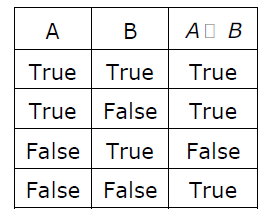# GATE | GATE-CS-2006 | Question 28

A logical binary relation □ ,is defined as follows:Let ~ be the unary negation (NOT) operator, with higher precedence than □.

Which one of the following is equivalent to A∧B ?

```(A) (~A □ B)
(B) ~(A □ ~B)
(C) ~(~A □ ~B)
(D) ~(~A □ B) ```

(A) A
(B) B
(C) C
(D) D